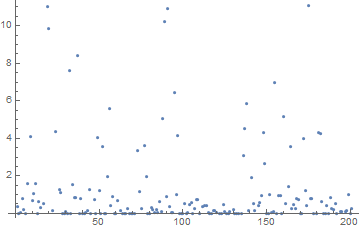Making public keys factorable with Rowhammer

The security of RSA encryption depends on the fact that the product of two large primes is difficult to factor. So if p and q are large primes, say 2048 bits each, then you can publish n = pq with little fear that someone can factor n to recover p and q.

But if you can change n by a tiny amount, you may make it much easier to factor. The Rowhammer attack does this by causing DRAM memory to flip bits.

To illustrate this on a small scale, let p = 251 and q = 643.  Then n = pq = 161393. If we flip the last bit of n we get m = 161392. Although n is hard to factor because it has no small factors, m is easy to factor, and in fact

161392 = 24 × 7 × 11 × 131.

For a larger example, I generated two 100-bit random primes in Mathematica:

p = 1078376712338123201911958185123
q = 1126171711601272883728179081277

and was able to factor n= pq in about 100 seconds. But Mathematica was able to factor n-1 in a third of a second.

So far we have looked at flipping the least significant bit. But Rowhammer isn’t that precise. It might flip some other bit.

If you flip any bit of a product of two large primes, you’re likely to get an easier factoring problem, though the difficulty depends on the number you start with and which bit you flip. To illustrate this, I flipped each of the bits one at a time and measured how long the result takes to factor.

The median time to factor n with one bit flipped was 0.4 seconds. Here’s a plot of the factoring times as a function of which bit was flipped.The plot shows about 80% of the data. Twenty percent of the time the value was above 11 seconds, and the maximum value was 74 seconds. So in every case flipping one bit made the factorization easier, usually quite a lot easier, but only a little easier in the worst case.

Related posts